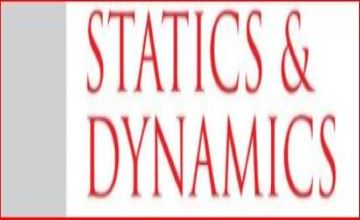Statics and Dynamics for UPSC

Ramanasri IAS Maths Optional is teaching Statics and Dynamics for UPSC. Ramanasri Sir is also teaching all the 13 Modules. For example from 1st Module Linear Algebra to Last i.e.,13th Module Mechanics & Fluid Dynamics. These Statics and Dynamics Module are Difficulty Level like Real Analysis, Modern Algebra, Mechanics and Fluid Dynamics. In Ramanasri IAS Ramanasri Sir is delivering Best Lectures for these all four Modules in addition to the rest of 9 Modules. The rest of the 9 Modules 5 Modules are very easy like Vector Analysis, Complex Analysis, Linear Programming, Numerical Analysis and Computer Programming, Calculus.
Medium Level Modules are Ordinary Differential Equations, Partial Differential Equations, Analytical Geometry.

In this Below we are giving Statics and Dynamics for UPSC Maths Syllabus. And also Statics and Dynamics for IFS or IFoS Maths Syllabus.

Statics and Dynamics for UPSC Maths Syllabus | IAS Maths Syllabus

Rectilinear motion, simple harmonic motion, motion in a plane, projectiles; constrained motion; Work and energy, conservation of energy; Kepler’s laws, orbits under central forces. Equilibrium of a system of particles; Work and potential energy, friction; common catenary; Principle of virtual work; Stability of equilibrium, the equilibrium of forces in three dimensions.

Statics and Dynamics for IFS Maths Syllabus | IFoS Maths Syllabus.

Dynamics, Statics and Hydro Statics :

Dynamics: Degree of freedom and constraints, rectilinear motion, simple harmonic motion, motion in a plane, projectiles, constrained motion, work and energy, conservation of energy, motion under impulsive forces, Kepler’s laws, orbits under central forces, the motion of the varying mass, motion under resistance.

Statics: Equilibrium of a system of particles, work, and potential energy, friction, common Catenary, the principle of virtual work, the stability of equilibrium, the equilibrium of forces in three dimensions.

Hydro Statics : The pressure of heavy fluids, the equilibrium of fluids under a given system of forces Bernoulli’s equation, the center of pressure, thrust on curved surfaces, the equilibrium of floating bodies, the stability of equilibrium, metacenter, the pressure of gases.

Statics and Dynamics Common topics for UPSC Maths Syllabus | IAS Maths Syllabus & IFS or IFoS Maths Syllabus

Dynamics : Rectilinear motion, Simple harmonic motion, motion in a plane, projectiles; constrained motion; Work and energy, conservation of energy; Kepler’s laws, orbits under central forces.

Statics: Equilibrium of a system of particles; Work and potential energy, friction; common catenary; Principle of virtual work; Stability of equilibrium, the equilibrium of forces in three dimensions.

List of topics of Statics and Dynamics for ONLY UPSC Maths Syllabus | IAS Maths Syllabus

No topics

List of topics of Statics and Dynamics for ONLY IFS Maths Syllabus | IFoS Maths Syllabus

Dynamics: Degree of freedom and constraints, motion under impulsive forces, the motion of the varying mass, motion under resistance.

Hydro Statics : The pressure of heavy fluids, the equilibrium of fluids under a given system of forces Bernoulli’s equation, the center of pressure, thrust on curved surfaces, the equilibrium of floating bodies, the stability of equilibrium, metacenter, the pressure of gases.

Click on this below links to know more details.

### Register Now

What are you waiting for ?